# Existence of anti-periodic solutions for second-order ordinary differential equations involving the Fučík spectrum

## Abstract

In this paper, we study the existence of anti-periodic solutions for a second-order ordinary differential equation. Using the interaction of the nonlinearity with the Fučík spectrum related to the anti-periodic boundary conditions, we apply the Leray-Schauder degree theory and the Borsuk theorem to establish new results on the existence of anti-periodic solutions of second-order ordinary differential equations. Our nonlinearity may cross multiple consecutive branches of the Fučík spectrum curves, and recent results in the literature are complemented and generalized.

## 1 Introduction and main results

In this paper, we study the existence of anti-periodic solutions for the following second-order ordinary differential equation:

$-{x}^{″}=f\left(t,x\right),$
(1.1)

where $f\in C\left({\mathbb{R}}^{2},\mathbb{R}\right)$, $f\left(t+\frac{T}{2},-s\right)=-f\left(t,s\right)$, $\mathrm{\forall }t,s\in \mathbb{R}$ and T is a positive constant. A function $x\left(t\right)$ is called an anti-periodic solution of (1.1) if $x\left(t\right)$ satisfies (1.1) and $x\left(t+\frac{T}{2}\right)=-x\left(t\right)$ for all $t\in \mathbb{R}$. Note that to obtain anti-periodic solutions of (1.1), it suffices to find solutions of the following anti-periodic boundary value problem:

$\left\{\begin{array}{c}{x}^{″}=-f\left(t,x\right),\hfill \\ {x}^{\left(i\right)}\left(0\right)=-{x}^{\left(i\right)}\left(\frac{T}{2}\right),\phantom{\rule{1em}{0ex}}i=0,1.\hfill \end{array}$
(1.2)

In what follows, we will consider problem (1.2) directly.

The problem of the existence of solutions of (1.1) under various boundary conditions has been widely investigated in the literature and many results have been obtained (see ). Usually, the asymptotic interaction of the ratio $\frac{f\left(t,s\right)}{s}$ with the Fučík spectrum of $-{x}^{″}$ under various boundary conditions was required as a nonresonance condition to obtain the solvability of equation (1.1). Recall that the Fučík spectrum of $-{x}^{″}$ with an anti-periodic boundary condition is the set of real number pairs $\left({\lambda }_{+},{\lambda }_{-}\right)\in {\mathbb{R}}^{2}$ such that the problem

$\left\{\begin{array}{c}-{x}^{″}={\lambda }_{+}{x}^{+}-{\lambda }_{-}{x}^{-},\hfill \\ {x}^{\left(i\right)}\left(0\right)=-{x}^{\left(i\right)}\left(\frac{T}{2}\right),\phantom{\rule{1em}{0ex}}i=0,1\hfill \end{array}$
(1.3)

has nontrivial solutions, where ${x}^{+}=max\left\{0,x\right\}$, ${x}^{-}=max\left\{0,-x\right\}$; while the concept of Fučík spectrum was firstly introduced in the 1970s by Fučík  and Dancer  independently under the periodic boundary condition. Since the work of Fonda , some investigation has been devoted to the nonresonance condition of (1.1) by studying the asymptotic interaction of the ratio $\frac{2F\left(t,s\right)}{{s}^{2}}$, where $F\left(t,s\right)={\int }_{0}^{s}f\left(t,\tau \right)\phantom{\rule{0.2em}{0ex}}d\tau$, with the spectrum of $-{x}^{″}$ under different boundary conditions; for instance, see  for the periodic boundary condition,  for the two-point boundary condition. Note that

$\underset{s\to ±\mathrm{\infty }}{lim inf}\frac{f\left(t,s\right)}{s}\le \underset{s\to ±\mathrm{\infty }}{lim inf}\frac{2F\left(t,s\right)}{{s}^{2}}\le \underset{s\to ±\mathrm{\infty }}{lim sup}\frac{2F\left(t,s\right)}{{s}^{2}}\le \underset{s\to ±\mathrm{\infty }}{lim sup}\frac{f\left(t,s\right)}{s},$

we can see that the conditions on the ratio $\frac{2F\left(t,s\right)}{{s}^{2}}$ are more general than those on the ratio $\frac{f\left(t,s\right)}{s}$. In fact, by using the asymptotic interaction of the ratio $\frac{2F\left(t,s\right)}{{s}^{2}}$ with the spectrum of $-{x}^{″}$, the ratio $\frac{f\left(t,s\right)}{s}$ can cross multiple spectrum curves of $-{x}^{″}$. In this paper, we are interested in the nonresonance condition on the ratio $\frac{2F\left(t,s\right)}{{s}^{2}}$ for the solvability of (1.1) involving the Fučík spectrum of $-{x}^{″}$ under the anti-periodic boundary condition.

Note that the study of anti-periodic solutions for nonlinear differential equations is closely related to the study of periodic solutions. In fact, since $f\left(t,s\right)=-f\left(t+\frac{T}{2},-s\right)=f\left(t+T,s\right)$, $x\left(t\right)$ is a T-periodic solution of (1.1) if $x\left(t\right)$ is a $\frac{T}{2}$-anti-periodic solution of (1.1). Many results on the periodic solutions of (1.1) have been worked out. For some recent work, one can see [25, 810, 17]. As special periodic solutions, the existence of anti-periodic solutions plays a key role in characterizing the behavior of nonlinear differential equations coming from some models in applied sciences. During the last thirty years, anti-periodic problems of nonlinear differential equations have been extensively studied since the pioneering work by Okochi . For example, in , anti-periodic trigonometric polynomials are used to investigate the interpolation problems, and anti-periodic wavelets are studied in . Also, some existence results of ordinary differential equations are presented in [17, 2124]. Anti-periodic boundary conditions for partial differential equations and abstract differential equations are considered in . For recent developments involving the existence of anti-periodic solutions, one can also see  and the references therein.

Denote by Σ the Fuc̆ík spectrum of the operator $-{x}^{″}$ under the anti-periodic boundary condition. Simple computation implies that $\mathrm{\Sigma }={\bigcup }_{m=1}^{+\mathrm{\infty }}{\mathrm{\Sigma }}_{m}$, where

It is easily seen that the set Σ can be seen as a subset of the Fuc̆ík spectrum of $-{x}^{″}$ under the corresponding Dirichlet boundary condition; one can see the definition of the set ${\mathrm{\Sigma }}_{2i+1}$, $i\in \mathbb{N}$, or Figure 1 in . Without loss of generality, we assume that ${\phi }_{m}$ is an eigenfunction of (1.3) corresponding to $\left({\lambda }_{+},{\lambda }_{-}\right)\in {\mathrm{\Sigma }}_{m}$ such that ${\phi }_{m}\left(0\right)=0$ and ${\phi }_{m}^{\prime }\left(0\right)=a\in \mathbb{R}\setminus \left\{0\right\}$. Denote ${\mathrm{\Sigma }}_{m,1}=\left\{\left({\lambda }_{+},{\lambda }_{-}\right)\in {\mathbb{R}}^{2}:\frac{\left(m+1\right)\pi }{\sqrt{{\lambda }_{+}}}+\frac{m\pi }{\sqrt{{\lambda }_{-}}}=\frac{T}{2},m\in {\mathbb{Z}}^{+}\right\}$ and ${\mathrm{\Sigma }}_{m,2}=\left\{\left({\lambda }_{+},{\lambda }_{-}\right)\in {\mathbb{R}}^{2}:\frac{m\pi }{\sqrt{{\lambda }_{+}}}+\frac{\left(m+1\right)\pi }{\sqrt{{\lambda }_{-}}}=\frac{T}{2},m\in {\mathbb{Z}}^{+}\right\}$. Then if $a>0$, we obtain only a one-dimensional function ${\phi }_{m}$, denoted by ${\phi }_{m,1}$, corresponding to the point $\left({\lambda }_{+},{\lambda }_{-}\right)\in {\mathrm{\Sigma }}_{m,1}$, and if $a<0$, we obtain only a one-dimensional function ${\phi }_{m}$, denoted by ${\phi }_{m,2}$, corresponding to the point $\left({\lambda }_{+},{\lambda }_{-}\right)\in {\mathrm{\Sigma }}_{m,2}$.

In this paper, together with the Leray-Schauder degree theory and the Borsuk theorem, we obtain new existence results of anti-periodic solutions of (1.1) when the nonlinearity $f\left(t,s\right)$ is asymptotically linear in s at infinity and the ratio $\frac{F\left(t,s\right)}{{s}^{2}}$ stays asymptotically at infinity in some rectangular domain between Fučík spectrum curves ${\mathrm{\Sigma }}_{m}$ and ${\mathrm{\Sigma }}_{m+1}$.

Our main result is as follows.

Theorem 1.1 Assume that $f\in C\left({\mathbb{R}}^{2},\mathbb{R}\right)$, $f\left(t+\frac{T}{2},-s\right)=-f\left(t,s\right)$. If the following conditions:

1. (i)

There exist positive constants ρ, ${C}_{1}$, M such that

$\rho \le \frac{f\left(t,s\right)}{s}\le {C}_{1},\phantom{\rule{1em}{0ex}}\mathrm{\forall }t\in \mathbb{R},\mathrm{\forall }|s|\ge M;$
(1.4)
2. (ii)

There exist connect subset $\mathrm{\Gamma }\subset {\mathbb{R}}^{2}\setminus \mathrm{\Sigma }$, constants ${p}_{1},{q}_{1},{p}_{2},{q}_{2}>0$ and a point of the type $\left(\lambda ,\lambda \right)\in {\mathbb{R}}^{2}$ such that

$\left(\lambda ,\lambda \right)\in \left[{p}_{1},{q}_{1}\right]×\left[{p}_{2},{q}_{2}\right]\subset \mathrm{\Gamma }$
(1.5)

and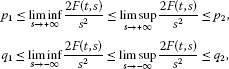hold uniformly for all $t\in \mathbb{R}$,

then (1.1) admits a $\frac{T}{2}$-anti-periodic solution.

In particular, if ${\lambda }_{+}={\lambda }_{-}$, then problem (1.3) becomes the following linear eigenvalue problem:

$\left\{\begin{array}{c}-{x}^{″}=\lambda x,\hfill \\ {x}^{\left(i\right)}\left(0\right)=-{x}^{\left(i\right)}\left(\frac{T}{2}\right),\phantom{\rule{1em}{0ex}}i=0,1.\hfill \end{array}$
(1.6)

Simple computation implies that the operator $-{x}^{″}$ with the anti-periodic boundary condition has a sequence of eigenvalues ${\lambda }_{m}=\frac{4{\left(2m-1\right)}^{2}{\pi }^{2}}{{T}^{2}}$, $m\in {\mathbb{Z}}^{+}$, and the corresponding eigenspace is two-dimensional.

Corollary 1.2 Assume that $f\in C\left({\mathbb{R}}^{2},\mathbb{R}\right)$, $f\left(t,s\right)=-f\left(t+\frac{T}{2},-s\right)$. If (1.4) holds and there exist constants p, q and $m\in {\mathbb{Z}}^{+}$ such that

$\frac{4{\left(2m-1\right)}^{2}{\pi }^{2}}{{T}^{2}}

holds uniformly for all $t\in \mathbb{R}$, then (1.1) admits a $\frac{T}{2}$-anti-periodic solution.

Remark It is well known that (1.1) has a $\frac{T}{2}$-anti-periodic solution if

$\underset{|s|\to +\mathrm{\infty }}{lim sup}\frac{f\left(t,s\right)}{s}\le {\sigma }_{1}<\frac{4{\pi }^{2}}{{T}^{2}}={\lambda }_{1},\phantom{\rule{1em}{0ex}}\mathrm{\forall }t\in \mathbb{R},$

for some ${\sigma }_{1}>0$ (see Theorem 3.1 in ), which implies that the ratio $\frac{f\left(t,s\right)}{s}$ stays at infinity asymptotically below the first eigenvalue ${\lambda }_{1}$ of (1.6). In this paper, this requirement on the ratio $\frac{f\left(t,s\right)}{s}$ can be relaxed to (1.4), with some additional restrictions imposed on the ratio $\frac{2F\left(t,s\right)}{{s}^{2}}$. In fact, the conditions relative to the ratios $\frac{f\left(t,s\right)}{s}$ and $\frac{2F\left(t,s\right)}{{s}^{2}}$ as in Theorem 1.1 and Corollary 1.2 may lead to that the ratio $\frac{f\left(t,s\right)}{s}$ oscillates and crosses multiple consecutive eigenvalues or branches of the Fučík spectrum curves of the operator $-{x}^{″}$. In what follows, we give an example to show this.

Denote ${\lambda }_{m}=\frac{4{\left(2m-1\right)}^{2}{\pi }^{2}}{{T}^{2}}$ for some positive integer $m\ge 1$. Define

$f\left(t,s\right)=cos\left(\frac{2\pi }{T}t\right)+\frac{{\lambda }_{m}+{\lambda }_{m+1}}{2}s+\left(\frac{{\lambda }_{m}+{\lambda }_{m+1}}{2}-\delta \right)scoss,\phantom{\rule{1em}{0ex}}\mathrm{\forall }t\in \mathbb{R},s\in \mathbb{R},$

where $\delta \in \left(0,\frac{{\lambda }_{1}}{100}\right)$. Clearly,

$\begin{array}{rcl}f\left(t+\frac{T}{2},-s\right)& =& cos\left[\frac{2\pi }{T}\left(t+\frac{T}{2}\right)\right]-\left[\frac{{\lambda }_{m}+{\lambda }_{m+1}}{2}s+\left(\frac{{\lambda }_{m}+{\lambda }_{m+1}}{2}-\delta \right)scoss\right]\\ =& -f\left(t,s\right).\end{array}$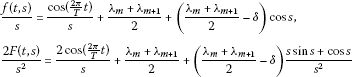for all $t\in \mathbb{R}$, $s\in \mathbb{R}$, which imply that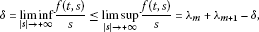(1.7)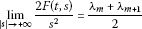(1.8)

for all $t\in \mathbb{R}$. It is obvious that (1.7) implies that the assumption (i) of Theorem 1.1 holds. Take ${p}_{1}=\frac{{\lambda }_{m}+{\lambda }_{m+1}}{2}-{\sigma }_{1}$, ${p}_{2}=\frac{{\lambda }_{m}+{\lambda }_{m+1}}{2}+{\sigma }_{1}$, ${q}_{1}=\frac{{\lambda }_{m}+{\lambda }_{m+1}}{2}-{\sigma }_{2}$, ${q}_{2}=\frac{{\lambda }_{m}+{\lambda }_{m+1}}{2}-{\sigma }_{2}$ such that $\left[{p}_{1},{p}_{2}\right]×\left[{q}_{1},{q}_{2}\right]\subset {\mathbb{R}}^{2}\setminus \mathrm{\Sigma }$. Then (1.8) implies that the assumption (ii) of Theorem 1.1 holds. Thus, by Theorem 1.1 we can obtain a $\frac{T}{2}$-anti-periodic solution of equation (1.1). Here the ratio $\frac{2F\left(t,s\right)}{{s}^{2}}$ stays at infinity in the rectangular domain $\left[{p}_{1},{p}_{2}\right]×\left[{q}_{1},{q}_{2}\right]$ between Fučík spectrum curves ${\mathrm{\Sigma }}_{m}$ and ${\mathrm{\Sigma }}_{m+1}$, while the ratio $\frac{f\left(t,s\right)}{s}$ can cross at infinity multiple Fučík spectrum curves ${\mathrm{\Sigma }}_{1},{\mathrm{\Sigma }}_{2},\dots ,{\mathrm{\Sigma }}_{m+1}$.

This paper is organized as follows. In Section 2, some necessary preliminaries are presented. In Section 3, we give the proof of Theorem 1.1.

## 2 Preliminaries

Assume that $T>0$. Define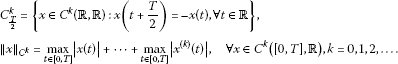For $x\in {C}_{\frac{T}{2}}^{k}$, we can write the Fourier series expansion as follows:

$x\left(t\right)=\sum _{i=0}^{\mathrm{\infty }}\left[{a}_{2i+1}cos\frac{2\pi \left(2i+1\right)t}{T}+{b}_{2i+1}sin\frac{2\pi \left(2i+1\right)t}{T}\right].$

Define an operator $J:{C}_{\frac{T}{2}}^{k}\to {C}_{\frac{T}{2}}^{k+1}$ by

$\begin{array}{rcl}\left(Jx\right)\left(t\right)& =& {\int }_{0}^{t}x\left(s\right)\phantom{\rule{0.2em}{0ex}}ds-\frac{T}{2\pi }\sum _{i=0}^{\mathrm{\infty }}\frac{{b}_{2i+1}}{2i+1}\\ =& \frac{T}{2\pi }\sum _{i=0}^{\mathrm{\infty }}\left[\frac{{a}_{2i+1}}{2i+1}sin\frac{2\pi \left(2i+1\right)t}{T}-\frac{{b}_{2i+1}}{2i+1}cos\frac{2\pi \left(2i+1\right)t}{T}\right].\end{array}$

Clearly,

$\frac{dJx\left(t\right)}{dt}=x\left(t\right),\phantom{\rule{2em}{0ex}}\left(Jx\right)\left(0\right)=-\frac{T}{2\pi }\sum _{i=0}^{\mathrm{\infty }}\frac{{b}_{2i+1}}{2i+1},$

which implies that

$\frac{{d}^{2}\left({J}^{2}x\left(t\right)\right)}{d{t}^{2}}=x\left(t\right).$
(2.1)

Furthermore, we obtain

$|Jx\left(t\right)|\le {\int }_{0}^{T}|x\left(s\right)|\phantom{\rule{0.2em}{0ex}}ds+\frac{T}{2\pi }\sum _{i=0}^{\mathrm{\infty }}\frac{|{b}_{2i+1}|}{2i+1}\le T{\parallel x\parallel }_{{C}^{k}}+\frac{T}{2\pi }{\left(\sum _{i=0}^{\mathrm{\infty }}{b}_{2i+1}^{2}\right)}^{\frac{1}{2}}{\left(\sum _{i=0}^{\mathrm{\infty }}\frac{1}{{\left(2i+1\right)}^{2}}\right)}^{\frac{1}{2}}.$

Note that

${\left(\sum _{i=0}^{\mathrm{\infty }}\frac{1}{{\left(2i+1\right)}^{2}}\right)}^{\frac{1}{2}}=\frac{\pi }{2\sqrt{2}},$

using the Parseval equality ${\int }_{0}^{T}{|x\left(s\right)|}^{2}\phantom{\rule{0.2em}{0ex}}ds=\frac{T}{2}{\sum }_{i=0}^{\mathrm{\infty }}\left[{a}_{2i+1}^{2}+{b}_{2i+1}^{2}\right]$, we get

$\begin{array}{rcl}|Jx\left(t\right)|& \le & T{\parallel x\parallel }_{{C}^{k}}+\frac{T}{4\sqrt{2}}\sum _{i=0}^{\mathrm{\infty }}\left[{a}_{2i+1}^{2}+{b}_{2i+1}^{2}\right]\\ \le & T{\parallel x\parallel }_{{C}^{k}}+\frac{T}{4\sqrt{2}}\frac{2}{T}{\int }_{0}^{T}{|x\left(s\right)|}^{2}\phantom{\rule{0.2em}{0ex}}ds\\ \le & \frac{5T}{4}{\parallel x\parallel }_{{C}^{k}},\phantom{\rule{1em}{0ex}}\mathrm{\forall }t\in \left[0,T\right],\end{array}$

which implies that the operator J is continuous. In view of the Arzela-Ascoli theorem, it is easy to see that J is completely continuous.

Denote by deg the Leray-Schauder degree. We need the following results.

Lemma 2.1 ([, p.58])

Let Ω be a bounded open region in a real Banach space X. Assume that $K:\overline{\mathrm{\Omega }}\to \mathbb{R}$ is completely continuous and $p\notin \left(I-K\right)\left(\partial \mathrm{\Omega }\right)$. Then the equation $\left(I-K\right)\left(x\right)=p$ has a solution in Ω if $deg\left(I-K,\mathrm{\Omega },p\right)\ne 0$.

Lemma 2.2 ([, Borsuk theorem, p.58])

Assume that X is a real Banach space. Let Ω be a symmetric bounded open region with $\theta \in \mathrm{\Omega }$. Assume that $K:\overline{\mathrm{\Omega }}\to \mathbb{R}$ is completely continuous and odd with $\theta \notin \left(I-K\right)\left(\partial \mathrm{\Omega }\right)$. Then $deg\left(I-K,\mathrm{\Omega },\theta \right)$ is odd.

## 3 Proof of Theorem 1.1

Proof of Theorem 1.1 Consider the following homotopy problem: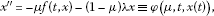(3.1)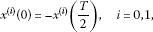(3.2)

where $\left(\lambda ,\lambda \right)\in \left[{p}_{1},{p}_{2}\right]×\left[{q}_{1},{q}_{2}\right]$, $\mu \in \left[0,1\right]$.

We first prove that the set of all possible solutions of problem (3.1)-(3.2) is bounded. Assume by contradiction that there exist a sequence of number $\left\{{\mu }_{n}\right\}\subset \left[0,1\right]$ and corresponding solutions $\left\{{x}_{n}\right\}$ of (3.1)-(3.2) such that

${\parallel {x}_{n}\parallel }_{{C}^{1}}\to +\mathrm{\infty }.$
(3.3)

Set ${z}_{n}=\frac{{x}_{n}}{{\parallel {x}_{n}\parallel }_{{C}^{1}}}$. Obviously, ${\parallel {z}_{n}\parallel }_{{C}^{1}}=1$ and ${z}_{n}$ satisfies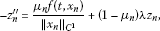(3.4)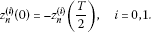(3.5)

By (1.4), (3.3) and the fact that f is continuous, there exist ${n}_{0}\in {\mathbb{Z}}^{+}$, ${C}_{1}>0$ such that

In view of ${\mu }_{n}\in \left[0,1\right]$, together with the choice of $\left(\lambda ,\lambda \right)$, it follows that there exists ${M}_{1}>0$ such that, for all $n\ge {n}_{0}$,

$|{z}_{n}^{″}\left(t\right)|\le {M}_{1},\phantom{\rule{1em}{0ex}}\mathrm{\forall }t\in \left[0,T\right].$

It is easily seen that $\left\{{z}_{n}\left(t\right)\right\}$ and $\left\{{z}_{n}^{\prime }\left(t\right)\right\}$ are uniformly bounded and equicontinuous on $\left[0,T\right]$. Then, using the Arzela-Ascoli theorem, there exist uniformly convergent subsequences on $\left[0,T\right]$ for $\left\{{z}_{n}\left(t\right)\right\}$ and $\left\{{z}_{n}^{\prime }\left(t\right)\right\}$ respectively, which are still denoted as $\left\{{z}_{n}\left(t\right)\right\}$ and $\left\{{z}_{n}^{\prime }\left(t\right)\right\}$, such that

$\underset{n\to \mathrm{\infty }}{lim}{z}_{n}\left(t\right)=z\left(t\right),\phantom{\rule{2em}{0ex}}\underset{n\to \mathrm{\infty }}{lim}{z}_{n}^{\prime }\left(t\right)={z}^{\prime }\left(t\right).$
(3.6)

Clearly, ${\parallel z\parallel }_{{C}^{1}}=1$. Since ${x}_{n}\left(t\right)$ is a solution of (3.1)-(3.2), for each n, we get

${\int }_{0}^{T}{x}_{n}\left(t\right)\phantom{\rule{0.2em}{0ex}}dt={\int }_{0}^{\frac{T}{2}}{x}_{n}\left(t\right)\phantom{\rule{0.2em}{0ex}}dt+{\int }_{0}^{\frac{T}{2}}{x}_{n}\left(t+\frac{T}{2}\right)\phantom{\rule{0.2em}{0ex}}dt=0,$

which implies that there exists ${t}_{n}\in \left[0,T\right]$ such that ${x}_{n}\left({t}_{n}\right)=0$. Then

$\underset{n\to \mathrm{\infty }}{lim}{z}_{n}\left({t}_{n}\right)=\underset{n\to \mathrm{\infty }}{lim}\frac{{x}_{n}\left({t}_{n}\right)}{{\parallel {x}_{n}\parallel }_{{C}^{1}}}=0.$
(3.7)

Owing to that the sequences $\left\{{t}_{n}\right\}$ and $\left\{{\mu }_{n}\right\}$ are uniformly bounded, there exist ${t}_{0}\in \left[0,T\right]$ and ${\mu }_{0}\in \left[0,1\right]$ such that, passing to subsequences if possible,

$\underset{n\to \mathrm{\infty }}{lim}{t}_{n}={t}_{0},\phantom{\rule{2em}{0ex}}\underset{n\to \mathrm{\infty }}{lim}{\mu }_{n}={\mu }_{0}.$
(3.8)

Multiplying both sides of (3.4) by ${z}_{n}^{\prime }\left(t\right)$ and integrating from ${t}_{n}$ to t, we get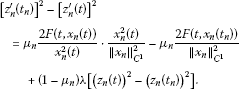Taking a superior limit as $n\to \mathrm{\infty }$, by (3.3) and (3.6)-(3.8), we obtain

${\left[{z}^{\prime }\left({t}_{0}\right)\right]}^{2}-{\left[{z}^{\prime }\left(t\right)\right]}^{2}={\mu }_{0}\underset{n\to \mathrm{\infty }}{lim sup}\frac{2F\left(t,{x}_{n}\left(t\right)\right)}{{x}_{n}^{2}\left(t\right)}\cdot {z}^{2}\left(t\right)+\left(1-{\mu }_{0}\right)\lambda {z}^{2}\left(t\right).$

By the assumption (ii) and the choice of λ, if $z\left(t\right)>0$, we have

${\left[{z}^{\prime }\left({t}_{0}\right)\right]}^{2}-{\left[{z}^{\prime }\left(t\right)\right]}^{2}\le {p}_{2}{z}^{2}\left(t\right).$

Similarly, we obtain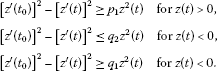Note that $z\left(t\right)\in {C}^{1}\left[0,T\right]$, the above inequalities can be rewritten as the following equivalent forms: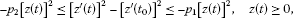(3.9)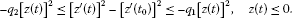(3.10)

It is easy to see that ${z}^{\prime }\left({t}_{0}\right)\ne 0$. In fact, if not, in view of (3.7)-(3.10), we get $z\left(t\right)=0$, ${z}^{\prime }\left(t\right)=0$, $\mathrm{\forall }t\in \left[0,T\right]$, which is contrary to ${\parallel z\parallel }_{{C}^{1}}=1$.

We claim that ${z}^{\prime }\left(t\right)$ has only finite zero points on $\left[0,T\right]$. In fact, if not, we may assume that there are infinitely many zero points $\left\{{\zeta }_{i}\right\}\subset \left[0,T\right]$ of ${z}^{\prime }\left(t\right)$. Without loss of generality, we assume that there exists ${\zeta }_{0}\in \left[0,T\right]$ such that ${lim}_{i\to \mathrm{\infty }}{\zeta }_{i}={\zeta }_{0}$. Letting $t={\zeta }_{i}$ in (3.9)-(3.10) and taking $i\to \mathrm{\infty }$, we can obtain that $z\left({\zeta }_{0}\right)\ne 0$. Without loss of generality, we assume that $z\left({\zeta }_{0}\right)>0$. Since $z\left(t\right)$ is continuous, there exist $\eta ,\delta >0$ such that $z\left(t\right)\ge \eta >0$, $\mathrm{\forall }t\in \left[{t}_{0}-\delta ,{t}_{0}+\delta \right]$. Then there exists ${n}_{1}>0$ such that, if $n>{n}_{1}$, we have

${z}_{n}\left(t\right)\ge \eta ,\phantom{\rule{1em}{0ex}}\mathrm{\forall }t\in \left[{t}_{0}-\delta ,{t}_{0}+\delta \right].$
(3.11)

Clearly, ${z}_{n}^{-}\left(t\right)=0$, $\mathrm{\forall }t\in \left[{t}_{0}-\delta ,{t}_{0}+\delta \right]$. Take ${\zeta }_{\ast },{\zeta }^{\ast }\in \left[{t}_{0}-\delta ,{t}_{0}+\delta \right]$ with ${\zeta }_{\ast }<{\zeta }^{\ast }$ such that ${z}^{\prime }\left({\zeta }^{\ast }\right)={z}^{\prime }\left({\zeta }_{\ast }\right)=0$. Integrating (3.4) from ${\zeta }_{\ast }$ to ${\zeta }^{\ast }$,

${z}_{n}^{\prime }\left({\zeta }_{\ast }\right)-{z}_{n}^{\prime }\left({\zeta }^{\ast }\right)={\mu }_{n}\frac{1}{{\parallel {x}_{n}\parallel }_{{C}^{1}}}{\int }_{{\zeta }_{\ast }}^{{\zeta }^{\ast }}f\left(t,{x}_{n}\left(s\right)\right)\phantom{\rule{0.2em}{0ex}}ds+\left(1-{\mu }_{n}\right){\int }_{{\zeta }_{\ast }}^{{\zeta }^{\ast }}\lambda {z}_{n}\left(s\right)\phantom{\rule{0.2em}{0ex}}ds.$
(3.12)

By (3.3), (3.11), we obtain

holds uniformly for $t\in \left[{\zeta }_{\ast },{\zeta }^{\ast }\right]$. Thus, using (1.4), we get

$\frac{f\left(t,{x}_{n}\left(t\right)\right)}{{x}_{n}\left(t\right)}\ge \rho ,\phantom{\rule{1em}{0ex}}\mathrm{\forall }t\in \left[{\zeta }_{\ast },{\zeta }^{\ast }\right],$

which implies that

$\frac{f\left(t,{x}_{n}\left(t\right)\right)}{{\parallel {x}_{n}\parallel }_{{C}^{1}}}=\frac{f\left(t,{x}_{n}\left(t\right)\right)}{{x}_{n}\left(t\right)}\cdot {z}_{n}\left(t\right)\ge \rho \cdot \eta >0,\phantom{\rule{1em}{0ex}}\mathrm{\forall }t\in \left[{\zeta }_{\ast },{\zeta }^{\ast }\right].$

Then, together with (3.6), (3.8) and (3.12), we obtain

$0\ge {\mu }_{0}\cdot \rho \cdot \eta \left({\zeta }^{\ast }-{\zeta }_{\ast }\right)+\left(1-{\mu }_{0}\right)\cdot \lambda \cdot \eta \left({\zeta }^{\ast }-{\zeta }_{\ast }\right)>0,$

Now, we show that (3.9)-(3.10) has only a trivial anti-periodic solution. In fact, if not, we assume that (3.9)-(3.10) has a nontrivial anti-periodic solution $\overline{z}\left(t\right)$. Without loss of generality, we assume ${t}_{0}=0$. Firstly, we consider the case that ${\overline{z}}^{\prime }\left(0\right)>0$. Assume that ${z}_{1}$, ${z}_{2}$ satisfy the following equations respectively: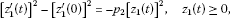(3.13)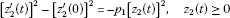(3.14)

with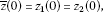(3.15)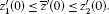(3.16)

Take ${t}_{1}$ as the first zero point of $\overline{z}\left(t\right)$ on $\left(0,T\right]$. Then by (3.13)-(3.16) it follows that

${z}_{1}\left(t\right)\le \overline{z}\left(t\right)\le {z}_{2}\left(t\right),\phantom{\rule{1em}{0ex}}\mathrm{\forall }t\in \left[0,{t}_{1}\right].$
(3.17)

In fact, by (3.15)-(3.16) and the fact that $\overline{z}$, ${z}_{1}$, ${z}_{2}$ are continuous differential, it is easy to see that there exists sufficiently small $ϵ\in \left(0,{t}_{1}\right)$ such that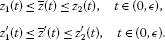If there is $\overline{t}\in \left(ϵ,{t}_{1}\right)$ such that $\overline{z}\left(\overline{t}\right)={z}_{1}\left(t\right)$, then comparing (3.9) with (3.13), we can obtain that ${\overline{z}}^{\prime }\left(\overline{t}\right)\ge {z}_{1}^{\prime }\left(\overline{t}\right)$, which implies that if $t>\overline{t}$, we have $\overline{z}\left(\overline{t}\right)\ge {z}_{1}\left(\overline{t}\right)$. Then $\overline{z}\left(t\right)\ge {z}_{1}\left(t\right)$ for $t\in \left(0,{t}_{1}\right]$. Similarly, we have $\overline{z}\left(t\right)\le {z}_{2}\left(t\right)$, $\mathrm{\forall }t\in \left[0,{t}_{1}\right]$. Hence, (3.17) holds.

Similarly, if ${z}_{1}$, ${z}_{2}$ satisfy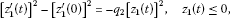(3.18)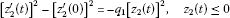(3.19)

and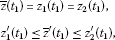then we obtain

${z}_{1}\left(t\right)\le \overline{z}\left(t\right)\le {z}_{2}\left(t\right),\phantom{\rule{1em}{0ex}}\mathrm{\forall }t\in \left[{t}_{1},{t}_{2}\right],$

where ${t}_{2}$ is the first zero point on $\left({t}_{1},T\right)$.

Since ${z}^{\prime }\left(t\right)$ has finite zero points, (3.13), (3.14), (3.18), (3.19) can be transformed into the following equations respectively: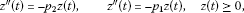(3.20)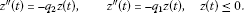(3.21)

Then there exist $A,B,C,D>0$ such that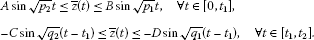It is easy to get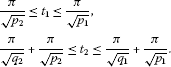Since $\overline{z}$ is anti-periodic and ${\overline{z}}^{\prime }\left(0\right)>0$, there exists $m\in {\mathbb{Z}}^{+}$ such that

$\frac{\left(m+1\right)\pi }{\sqrt{{q}_{2}}}+\frac{m\pi }{\sqrt{{p}_{2}}}\le {t}_{m}=\frac{T}{2}\le \frac{\left(m+1\right)\pi }{\sqrt{{q}_{1}}}+\frac{m\pi }{\sqrt{{p}_{1}}},$

which implies that there exists a real number pair $\left({p}^{\ast },{q}^{\ast }\right)\in \left[{p}_{1},{p}_{2}\right]×\left[{q}_{1},{q}_{2}\right]$ such that

$\frac{\left(m+1\right)\pi }{\sqrt{{q}^{\ast }}}+\frac{m\pi }{\sqrt{{p}^{\ast }}}=\frac{T}{2}.$
(3.22)

On the other hand, in view of the assumption (ii), by the definition of Σ and $\left({p}^{\ast },{q}^{\ast }\right)\in \left[{p}_{1},{p}_{2}\right]×\left[{q}_{1},{q}_{2}\right]$, it follows that

$\frac{\left(m+1\right)\pi }{\sqrt{{p}^{\ast }}}+\frac{m\pi }{\sqrt{{q}^{\ast }}}\ne \frac{T}{2},\phantom{\rule{1em}{0ex}}\mathrm{\forall }m\in {\mathbb{Z}}^{+},$

which is contrary to (3.22).

If ${\overline{z}}^{\prime }\left(0\right)<0$, then by the assumption (ii), we can obtain a contradiction using similar arguments.

In a word, we can see that there exists $C>0$ independent of μ such that

${\parallel x\parallel }_{{C}^{1}}\le C.$
(3.23)

Set

$\mathrm{\Omega }=\left\{x\in {C}_{\frac{T}{2}}^{1}:{\parallel x\parallel }_{{C}^{1}}

Clearly, Ω is a bounded open set in ${C}_{\frac{T}{2}}^{1}$. Note that, for $x\in {C}_{\frac{T}{2}}^{1}$, using the assumption on f, we obtain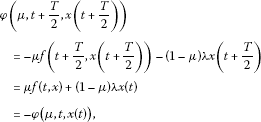which implies that $\phi \in {C}_{\frac{T}{2}}^{0}$.

Define ${G}_{\mu }:\overline{\mathrm{\Omega }}\to {C}_{\frac{T}{2}}^{2}$ by

${G}_{\mu }\left(x\left(t\right)\right)={J}^{2}\phi \left(\mu ,t,x\left(t\right)\right).$

Clearly, ${G}_{\mu }$ is completely continuous, and by (2.1) and (3.1) it follows that the fixed point of ${G}_{1}$ in $\overline{\mathrm{\Omega }}$ is the anti-periodic solution of problem (1.1). Define the homotopy $H:\overline{\mathrm{\Omega }}×\left[0,1\right]\to {C}_{\frac{T}{2}}^{1}$ as follows:

$H\left(x,\mu \right)=x-{G}_{\mu }\left(x\right).$

In view of (3.23), it follows that

$H\left(x,\mu \right)\ne 0,\phantom{\rule{1em}{0ex}}\mathrm{\forall }\left(x,\mu \right)\in \partial \mathrm{\Omega }×\left[0,1\right].$

Hence,

$deg\left(I-{G}_{1},\mathrm{\Omega },0\right)=deg\left(I-{G}_{0},\mathrm{\Omega },0\right).$

Note that the operator ${G}_{0}$ is odd. By Lemma 2.2 it follows that $deg\left(I-{G}_{0},\mathrm{\Omega },0\right)\ne 0$. Thus,

$deg\left(I-{G}_{1},\mathrm{\Omega },0\right)\ne 0.$

Now, using Lemma 2.1, we can see that (1.2) has a solution and hence (1.1) has a $\frac{T}{2}$-anti-periodic solution. The proof is complete. □

## References

1. Bravo JL, Torres PJ: Periodic solutions of a singular equation with indefinite weight. Adv. Nonlinear Stud. 2010, 10: 927-938.

2. Chu J, Fan N, Torres PJ: Periodic solutions for second order singular damped differential equations. J. Math. Anal. Appl. 2012, 388: 665-675. 10.1016/j.jmaa.2011.09.061

3. Chu J, Torres PJ: Applications of Schauder’s fixed point theorem to singular differential equations. Bull. Lond. Math. Soc. 2007, 39: 653-660. 10.1112/blms/bdm040

4. Chu J, Torres PJ, Zhang M: Periodic solutions of second order non-autonomous singular dynamical systems. J. Differ. Equ. 2007, 239: 196-212. 10.1016/j.jde.2007.05.007

5. Chu J, Zhang Z: Periodic solutions of singular differential equations with sign-changing potential. Bull. Aust. Math. Soc. 2010, 82: 437-445. 10.1017/S0004972710001607

6. Fonda A: On the existence of periodic solutions for scalar second order differential equations when only the asymptotic behaviour of the potential is known. Proc. Am. Math. Soc. 1993, 119: 439-445. 10.1090/S0002-9939-1993-1154246-4

7. Habets P, Omari P, Zanolin F: Nonresonance conditions on the potential with respect to the Fučík spectrum for the periodic boundary value problem. Rocky Mt. J. Math. 1995, 25: 1305-1340. 10.1216/rmjm/1181072148

8. Halk R, Torres PJ: On periodic solutions of second-order differential equations with attractive-repulsive singularities. J. Differ. Equ. 2010, 248: 111-126. 10.1016/j.jde.2009.07.008

9. Halk R, Torres PJ, Zamora M: Periodic solutions of singular second order differential equations: the repulsive case. Topol. Methods Nonlinear Anal. 2012, 39: 199-220.

10. Liu W, Li Y: Existence of 2 π -periodic solutions for the non-dissipative Duffing equation under asymptotic behaviors of potential function. Z. Angew. Math. Phys. 2006, 57: 1-11.

11. Omari P, Zanolin F: Nonresonance conditions on the potential for a second-order periodic boundary value problem. Proc. Am. Math. Soc. 1993, 117: 125-135. 10.1090/S0002-9939-1993-1143021-2

12. Tomiczek P: Potential Landesman-Lazer type conditions and the Fučík spectrum. Electron. J. Differ. Equ. 2005., 2005: Article ID 94

13. Zhang M: Nonresonance conditions for asymptotically positively homogeneous differential systems: the Fučík spectrum and its generalization. J. Differ. Equ. 1998, 145: 332-366. 10.1006/jdeq.1997.3403

14. Fučík S: Boundary value problems with jumping nonlinearities. Čas. Pěst. Mat. 1976, 101: 69-87.

15. Dancer EN: Boundary-value problems for weakly nonlinear ordinary differential equations. Bull. Aust. Math. Soc. 1976, 15: 321-328. 10.1017/S0004972700022747

16. Marcos A: Nonresonance conditions on the potential for a semilinear Dirichlet problem. Nonlinear Anal. 2009, 70: 335-351. 10.1016/j.na.2007.12.006

17. Gao E, Song S, Zhang X: Solving singular second-order initial/boundary value problems in reproducing kernel Hilbert space. Bound. Value Probl. 2012., 2012: Article ID 3

18. Okochi H: On the existence of periodic solutions to nonlinear abstract parabolic equations. J. Math. Soc. Jpn. 1988, 40: 541-553. 10.2969/jmsj/04030541

19. Delvos FJ, Knoche L: Lacunary interpolation by antiperiodic trigonometric polynomials. BIT Numer. Math. 1999, 39: 439-450. 10.1023/A:1022314518264

20. Chen H: Antiperiodic wavelets. J. Comput. Math. 1996, 14: 32-39.

21. Chen Y: Note on Massera’s theorem on anti-periodic solution. Adv. Math. Sci. Appl. 1999, 9: 125-128.

22. Chen T, Liu W, Yang C: Antiperiodic solutions for Liénard-type differential equation with p -Laplacian operator. Bound. Value Probl. 2010., 2010: Article ID 194824

23. Chen T, Liu W, Zhang J, Zhang H: Anti-periodic solutions for higher-order nonlinear ordinary differential equations. J. Korean Math. Soc. 2010, 47: 573-583. 10.4134/JKMS.2010.47.3.573

24. Chen Y, Nieto JJ, O’Regan D: Anti-periodic solutions for fully nonlinear first-order differential equations. Math. Comput. Model. 2007, 46: 1183-1190. 10.1016/j.mcm.2006.12.006

25. Aizicovici S, McKibben M, Reich S: Anti-periodic solutions to nonmonotone evolution equations with discontinuous nonlinearities. Nonlinear Anal. 2001, 43: 233-251. 10.1016/S0362-546X(99)00192-3

26. Chen Y, Nieto JJ, O’Regan D: Anti-periodic solutions for evolution equations associated with maximal monotone mappings. Appl. Math. Lett. 2011, 24: 302-307. 10.1016/j.aml.2010.10.010

27. Chen Y, O’Regan D, Agarwal RP: Anti-periodic solutions for semilinear evolution equations in Banach spaces. J. Appl. Math. Comput. 2012, 38: 63-70. 10.1007/s12190-010-0463-y

28. Ji S: Time-periodic solutions to a nonlinear wave equation with periodic or anti-periodic boundary conditions. Proc. R. Soc. Lond. Ser. A 2009, 465: 895-913. 10.1098/rspa.2008.0272

29. Ji S, Li Y: Time periodic solutions to one dimensional wave equation with periodic or anti-periodic boundary conditions. Proc. R. Soc. Edinb., Sect. A, Math. 2007, 137: 349-371. 10.1017/S0308210505001174

30. Liu Z: Anti-periodic solutions to nonlinear evolution equations. J. Funct. Anal. 2010, 258: 2026-2033. 10.1016/j.jfa.2009.11.018

31. Nakao M: Existence of anti-periodic solution for the quasilinear wave equation with viscosity. J. Math. Anal. Appl. 1996, 204: 754-764. 10.1006/jmaa.1996.0465

32. N’Guérékata GM, Valmorin V: Antiperiodic solutions of semilinear integrodifferential equations in Banach spaces. Appl. Math. Comput. 2012, 218: 11118-11124. 10.1016/j.amc.2012.05.005

33. Anahtarci B, Djakov P: Refined asymptotics of the spectral gap for the Mathieu operator. J. Math. Anal. Appl. 2012, 396: 243-255. doi:10.1016/j.jmaa.2012.06.019 10.1016/j.jmaa.2012.06.019

34. Ahmad B, Nieto JJ: Existence of solutions for impulsive anti-periodic boundary value problems of fractional order. Taiwan. J. Math. 2011, 15: 981-993.

35. Pan L, Cao J: Anti-periodic solution for delayed cellular neural networks with impulsive effects. Nonlinear Anal. 2011, 12: 3014-3027.

36. Deimling K: Nonlinear Functional Analysis. Springer, New York; 1985.

## Acknowledgements

The authors sincerely thank Prof. Yong Li for his instructions and many invaluable suggestions. This work was supported financially by NSFC Grant (11101178), NSFJP Grant (201215184), and the 985 Program of Jilin University.

## Author information

Authors

### Corresponding author

Correspondence to Xiaojun Chang.

### Competing interests

The authors declare that they have no competing interests.

### Authors’ contributions

All authors read and approved the final manuscript.

## Rights and permissions

Reprints and Permissions

Zhao, X., Chang, X. Existence of anti-periodic solutions for second-order ordinary differential equations involving the Fučík spectrum. Bound Value Probl 2012, 149 (2012). https://doi.org/10.1186/1687-2770-2012-149

• Accepted:

• Published:

• DOI: https://doi.org/10.1186/1687-2770-2012-149

### Keywords

• anti-periodic solutions
• Fučík spectrum
• Leray-Schauder degree theory
• Borsuk theorem